# DAV Class 7 Maths Chapter 3 Brain Teasers Solutions

The DAV Class 7 Maths Book Solutions Pdf and DAV Class 7 Maths Chapter 3 Brain Teasers Solutions of Rational Numbers as Decimals offer comprehensive answers to textbook questions.

## DAV Class 7 Maths Ch 3 Brain Teasers Solutions

Question 1.
A. Tick (✓) the correct option:
(i) 0.225 expressed as a rational number is
(a) $$\frac{1}{4}$$
(b) $$\frac{45}{210}$$
(c) $$\frac{9}{40}$$
(d) $$\frac{225}{999}$$
(c) $$\frac{9}{40}$$

0.225 = $$\frac{225}{1000}=\frac{9}{40}$$
Hence, (c) is the correct answer.

(ii) A rational number $$\frac{p}{q}$$ can be expressed as a terminating decimal if q ha no factor other than:
(a) 2, 3
(b) 2, 5
(c) 3, 5
(d) 2, 3, 5
(b) 2, 5

If the denominator (q) of a rational number ($$\frac{p}{q}$$) is the standard form has 2 or 5 or both only prime factors, then it can be represented as a terminating decimal
Hence, (b) is the correct answer

(iii) -7 $$\frac{8}{100}$$ expressed as a decimal number is:
(a) -7.800
(b) 7.008
(c) -7.008
(d) -7.08
(d) -7.08

-7$$\frac{8}{100}=\frac{-708}{100}$$ = -7.08
Hence, (d) is the correct answer.

(iv) 4.013$$\overline{25}$$ is equal to
(a) 4.013252525…….
(b) 4.01325555….
(c) 4.0132501325……
(d) 4.0130132525……..
(a) 4.013252525…….

4.013$$\overline{25}$$ = 4.013252525…….
Hence, (a) is the correct answer.

(v) The quotient when 0.00639 is divided by
(a) 3
(b) 0.3
(c) 0.03
(d) 0.003
(c) 0.03

$$\frac{0.00639}{0.213}=\frac{639}{100000} \times \frac{1000}{213}$$
= $$\frac{3}{100}$$ = 0.03
Hence, (c) is the correct answer.(i) Without actual division, determine if is terminating or non-terminating decimal number.
The denominator of $$\frac{-28}{250}$$ is 250.
250 = 2 × 5 × 5 × 5.
The prime factors of 250 are 2 and 5. But, the rational number is not in its lowest form.
In fact, $$\frac{-28}{250}=\frac{-14 \times 2}{125 \times 2}=\frac{-14}{125}$$, whose denominator 125 = 5 x 5 x 5
Therefore, $$\frac{-28}{250}$$ has a terminating decimal representation.

(ii) Convert $$\frac{-113}{7}$$ to decimals.
To represent $$\frac{-113}{7}$$ as a decimal, we divide 113 by 7.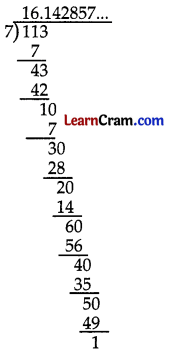∴ $$\frac{-113}{7}$$ = 16.142857…… = 16.$$\overline{142857}$$
$$\frac{-113}{7}$$ in decimal form is represented as 16.$$\overline{142857}$$
Therefore, $$\frac{-113}{7}$$ = 16.$$\overline{142857}$$

(iii) What should be subtracted from -15.834 to get 3.476?
Let x is subtracted from -15.834 to get 3.476
∴ -15.834 – x = 3.476
⇒ x = -15.834 – 3.476
⇒ x = -19.310
Therefore, -19.310 should be subtracted from – 15.834 to get 3.476

(iv) Express 4.82 as rational number in standard form.
4.82 = $$\frac{482}{100}=\frac{241}{50}$$

(v) Find the value of 16.016 ÷ 0.4.
16.016 ÷ 0.4
= $$\frac{16.016}{0.4}=\frac{16016}{1000} \times \frac{10}{4}=\frac{4004}{100}$$ = 40.04

Question 2.
Convert the following rational numbers into decimals:
(i) $$\frac{259}{3}$$∴ $$\frac{259}{3}$$ = 86.333 … = 86.3̄

(ii) $$\frac{19256}{11}$$∴ $$\frac{19256}{11}$$ = 175.5454…….
= 175.$$\overline{54}$$

(iii) $$\frac{15735}{80}$$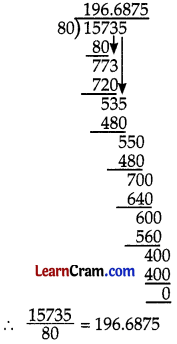(iv) $$\frac{27}{7}$$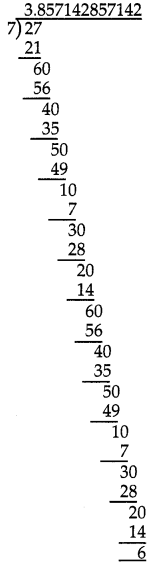∴ $$\frac{27}{7}$$ = 3.857142857142 = 3.$$\overline{857142}$$

(v) $$\frac{758}{1250}$$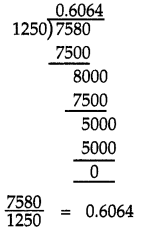(vi) $$\frac{15625}{12}$$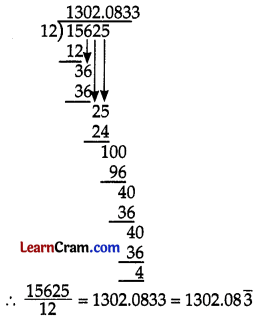Question 3.
Find the decimal representation of the following rational numbers:
(i) $$\frac{-12}{13}$$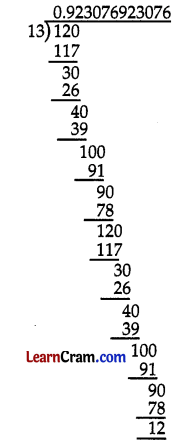∴ $$\frac{-12}{13}$$ = -0.923076923076….. = -0.$$\overline{923076}$$

(ii) $$\frac{-1525}{50}$$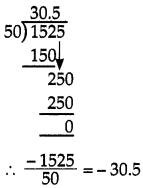(iii) $$\frac{-127}{7}$$Hence $$\frac{-127}{7}$$ = -18.142857142857…… = -18.$$\overline{142857}$$

(iv) $$-\frac{539}{80}$$∴ Hence $$-\frac{539}{80}$$ = -6.7375

Question 4.
Simplify the following expressions:
(i) 3.2 + 16.09 + 26.305 – 1.232
3.2 + 16.09 + 26.305 -1.232
= 3.200 +16.090 + 26.305 -1.232
= 45.595-1.232
= 44.363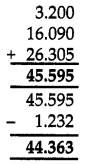(ii) -5.7 + 13.20 – 15.009 + 0.02
-5.7 + 13.20-15.009 + 0.02
= – 5.700 + 13.200 – 15.009 + 0.020
= (13.200 – 5.700) + (0 .020 -15.009)
= 7.500 + (-14.989)
= 7.500-14.989
= -7.489

(iii) (0.357 + 0.96) – (3.25 – 2.79)
(0.357 + 0.96) – (3.25 – 2.79)
= (0.357 + 0.960) – (3.250 – 2.790)
= 1.317-0.460
= 0.857

(iv) 15 + 2.57 – 23.07 – 5.003
15 + 2.57 – 23.07 – 5.003
= 15.000 + 2.570 – 23.070 – 5.003
= 17.570 – 28.073
= -10.503

Question 5.
Without actual division, determine which of the following rational numbers have a terminating decimal representation.
(i) $$\frac{327}{125}$$
125 = 5 × 5 × 5
= 53 × 20
∴ $$\frac{327}{125}$$ is a terminating decimal

(ii) $$\frac{99}{800}$$
800 = 32 × 25
= 25 × 52
∴ $$\frac{99}{800}$$ is a terminating decimal

(iii) $$\frac{17}{1250}$$
1250 = 54 × 21
∴ $$\frac{17}{1250}$$ is a terminating decimal

(iv) $$\frac{29}{200}$$
200 = 25 × 8
= 52 × 23
∴ $$\frac{29}{200}$$ is a terminating decimal

(v) $$\frac{135}{1625}$$
$$\frac{135}{1625}=\frac{135 \div 5}{1625+5}=\frac{27}{325}$$
325 = 5 × 5 × 13
It is not in the form of 5n × 2m
∴ $$\frac{135}{1625}$$ is a non – terminating decimal

(vi) $$\frac{1276}{680}$$
$$\frac{1276}{680}=\frac{1276 \div 4}{680 \div 4}=\frac{319}{170}$$
170 = 2 × 5 × 17
It is not in the form of 5m × 2n
∴ $$\frac{1276}{680}$$ is a non – terminating decimal

(vii) $$\frac{22}{190}$$
$$\frac{22}{190}=\frac{22 \div 2}{190 \div 2}=\frac{11}{95}$$
95 = 5 × 19
It is not in the form of 5m × 2n
∴ $$\frac{22}{190}$$ is a non – terminating decimal

(viii) $$\frac{11}{750}$$
750 = 3 × 5 × 5 × 5 × 2
It is not in the form of 5m × 2n
∴ $$\frac{11}{750}$$ is a non – terminating decimalQuestion 6.
Simplify the following and express the result as decimals.
(i) 2.7 × 1.5 × 2.12.7 × 1.5 × 2.1
= 4.05 × 2.1
= 8.505

(ii) 12 × 13.6 × 0.25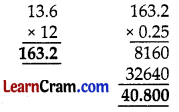12 × 13.6 × 0.25
= 163. 2 × 0.25
= 40.800
= 40.8

(iii) 3.25 × 72.6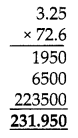3.25 × 72.6
= 231.95

(iv) (156.25 ÷ 0.0251 × 0.02 – 5.2
(156.25 ÷ 0.025) × 0.02 – 5.2
= $$\frac{156.25}{0.025}$$ × 0.02 – 5.2
= $$\frac{156250}{25}$$ × 0.02 – 5.20
= 6250 × 0.02 – 5.20
= 125.00 – 5.20
= 125 – 5.20
= 119.80
= 119.8

(v) (75.05 ÷ 0.05) × 0.001 + 2.351
(75.05 ÷ 0.05) × 0.001 + 2.351
= $$\frac{75.05}{0.05}$$ × 0.001 + 2.351
= $$\frac{7505}{5}$$ × 0.001 + 2.351
= 1501 × 0.001 + 2.351
= 1.501 + 2.351
= 3.852

Question 7.
Simplify and express the result as a rational number in its lowest form,
(i) 3.125 + 0.125 + 0.50 – 0.225
3.125 + 0.125 + 0.50 – 0.225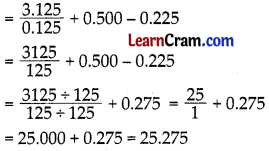(ii) $$\frac{0.4 \times 0.04 \times 0.005}{0.1 \times 10 \times 0.001}-\frac{1}{2}+\frac{1}{5}$$(iii) $$\frac{0.144 \div 1.2}{0.016 \div 0.02}+\frac{7}{5}-\frac{21}{8}$$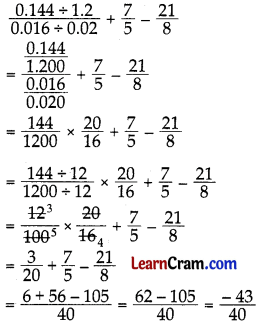### DAV Class 7 Maths Chapter 3 HOTS

Question 1.
Perimeter of a rectangle is 2.4 m less than $$\frac{2}{5}$$ of the perimeter of a square. If the perimeter of the square is 40 m, find the length and breadth of the rectangle give that breadth is $$\frac{1}{3}$$ of the length.
Let the length and breadth of the rectangle be l and b respectively.
∴ Perimeter of rectangle = 2(1 + b)
According to the question,
2(l + b) = $$\frac{2}{5}$$ (40) – 2.4
⇒ 2(l + b) = 2(8) – 2.4
⇒ 2(l + b) = 16 – 2.4
⇒ 2(l + b) = 13.6 …(1)

Also, b = $$\frac{1}{3}$$l [Given]
⇒ 3 b = l
⇒ l = 3b
Putting l = 3b in equation (1), we get
2(3 b + b) = 13.6
⇒ 2(4 b) = 13.6
⇒ 8b = 13.6
⇒ b = $$\frac{13.6}{8}$$
⇒ b = 1.7 m
∴ l = 3b
⇒ l = 3 × 1.7 m
= 5.1 m
Therefore, the length and breadth of the rectangle are 5.1 m and 1.7 m respectively.

### DAV Class 7 Maths Chapter 3 Enrichment Questions

Question 1.
There is an interesting pattern in the following: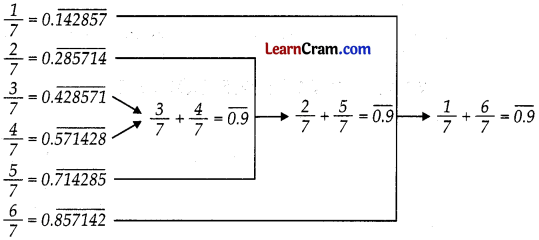You will question why the left hand side in each case is 1, but, the right hand side is 0.9 ? (You will learn about this in higher classes).
Notice they all have only the digits 142857, each starting with a different digit but in the same order.
Try finding out the repeating part of the decimal for $$\frac{1}{13}$$. What do you notice?
To represent $$\frac{1}{13}$$ asa decimal, we divide 1 by 13.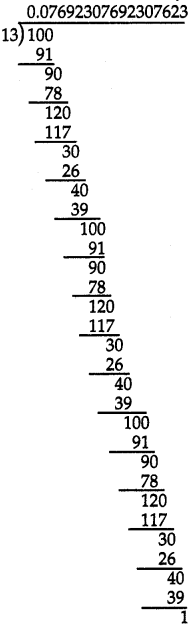∴ $$\frac{1}{13}$$ = 0.076923… = 0.$$\overline{076923}$$
Here, we have remainder as 1 which is just the same as the dividend. Therefore, after 3, the same digits, i.e., 0, 7, 6, 9, 2, 3 will keep on repeating again and again in the quotient.

Question 1.
Convert the following in the decimal form.
(i) $$\frac{-3}{125}$$
$$\frac{-3}{125}=\frac{-3 \times 8}{125 \times 8}$$
= $$\frac{-24}{1000}$$
= -0.024

(ii) $$\frac{4}{25}$$
$$\frac{4}{25}=\frac{4 \times 4}{25 \times 4}$$
= $$\frac{16}{100}$$
= -0.16

(iii) $$\frac{-7}{80}$$
$$\frac{-7}{80}=\frac{-7 \times 125}{80 \times 125}$$
= $$\frac{-875}{10000}$$
= -0.0875

(iv) $$\frac{-12}{5}$$
$$\frac{-12}{5}=\frac{-12 \times 2}{5 \times 2}$$
= $$\frac{-24}{10}$$
= -2.4

Question 2.
Express the following rational numbers as decimals by using long division method.
(i) $$\frac{629}{125}$$∴ $$\frac{629}{125}$$ = 5.032

(ii) $$\frac{317}{80}$$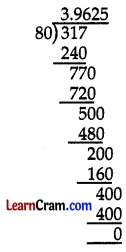∴ $$\frac{317}{80}$$ = 3.9625

Question 3.
Express the following rational numbers as decimals by using long division method.
(i) $$\frac{-23}{7}$$∴ $$\frac{-23}{7}$$ = -3.285714285714 ………… = -3.$$\overline{285714}$$

(ii) $$\frac{-37}{4}$$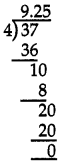∴ $$\frac{-37}{4}$$ = 9.25

(iii) $$\frac{62}{3}$$∴ $$\frac{62}{3}$$ = 20.6̄

(iv) $$\frac{523}{15}$$∴ $$\frac{523}{15}$$ = 34.866.. = 34.86̄

Question 4.
Without actual division find which of the following rational numbers have terminating decimal representation?
(i) $$\frac{-17}{130}$$
$$\frac{-17}{130}$$, 130 = 2 × 5 × 13
Here prime factors are not in the form 2m × 5n
∴ $$\frac{-17}{130}$$ is a non-terminating decimal.

(ii) $$\frac{21}{128}$$
$$\frac{21}{128}$$, 128 = 2 × 2 × 2 × 2 × 2 × 2
= 27 × 5n
∴ $$\frac{21}{128}$$ is a terminating decimal.

(iii) $$\frac{35}{216}$$
$$\frac{35}{216}$$, 216 = 2 × 2 × 2 × 3 × 3 × 3
= 23 × 33
∴ $$\frac{35}{216}$$ is a not a terminating decimal.

(iv) $$\frac{31}{200}$$
$$\frac{31}{200}$$, 200 = 2 × 2 × 2 × 5 × 5 = 23 × 52
Here the prime factors of 200 are in the form of 2m × 5n
∴ It is a terminating decimal.Question 5.
Express the following rational numbers in the form of $$\frac{p}{q}$$.
(i) 0.08
0.08 = $$\frac{8}{100}=\frac{8 \div 4}{100 \div 4}$$
= $$\frac{2}{25}$$

(ii) -0.16
-0.16 = $$\frac{-16}{100}=\frac{-16 \div 4}{100 \div 4}$$
= $$\frac{-4}{25}$$

(iii) -24.25
-24.25 = $$\frac{-2425}{100}=\frac{-2425 \div 25}{100 \div 25}$$
= $$\frac{-97}{4}$$

(iv) -0.25
-0.25 = $$\frac{-25}{100}=\frac{-25 \div 25}{100 \div 25}$$
= $$\frac{-1}{4}$$

Question 6.
Simplify: $$\frac{2}{3}+\frac{31}{48}-\frac{16}{9}+\frac{1}{12}$$
L.C.M of 3, 48, 9, 12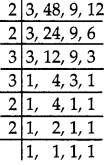= 2 × 2 × 3 × 3 × 2 × 2 = 144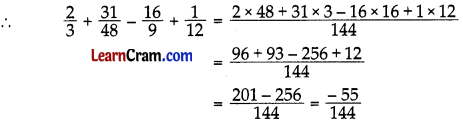Question 7.
Evaluate: 215.3 – 14.003 + 0.02 – 0.005
215.3 – 14.003 + 0.02 – 0.005
= 215.300 – 14.003 + 0.020 – 0.005
= 215.300 + 0.020 – 14.003 – 0.005
= 215.320 -14.008
= 201.312

Question 8.
Simplify the following and express the result in standard form.
(i) $$\frac{3}{4} \div \frac{7}{8} \times \frac{5}{16}+\frac{4}{5}-\frac{1}{3}$$(ii) $$\frac{0.001 \times 0.12}{3.50 \times 0.36}$$
$$\frac{0.001 \times 0.12}{3.50 \times 0.36}=\frac{0.00012}{1.2600}$$
⇒ $$\frac{0.00012}{1.26000}=\frac{12}{126000}$$
⇒ $$\frac{12 \div 12}{126000 \div 12}=\frac{1}{10500}$$Question 9.
Simplify the following expressions.
(i) (75.05 + 0.05) × 0.015 + 6.023
(75.05 + 0.05) × 0.015 + 6.023= 1501 × 0.015 + 6.023
= 22515 + 6.023
= 28.538

(ii) (30.05 + 0.01) + 0.005 + 2.005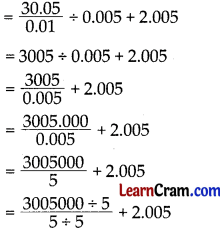= 601000 + 2.005
= 601002.005

Question 10.
Evaluate the following:
(i) 43.7 – 13 – 10.025 + 3.18